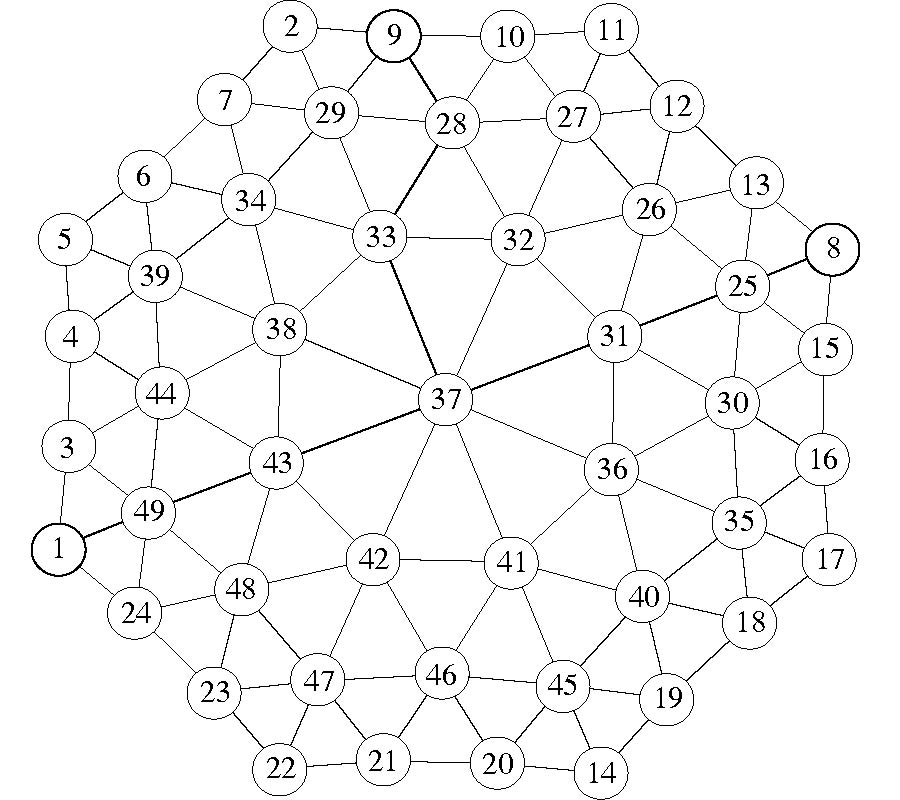# Vol. 9

2009-06-12

#### An Improved Algorithm for Matrix Bandwidth and Profile Reduction in Finite Element Analysis

By Qing Wang and Xiao-Wei Shi
Progress In Electromagnetics Research Letters, Vol. 9, 29-38, 2009
doi:10.2528/PIERL09042305

## Abstract

In finite element analysis, methods for the solution of sparse linear systems of equations usually start out with reordering the coefficient matrix to reduce its bandwidth or profile. The location of pseudo-peripheral nodes is an important factor in the bandwidth and profile reduction algorithm. This paper presents a heuristic parameter, called the "width-depth ratio" and denoted by κ. With such a parameter, suitable pseudo-peripheral nodes could be found; the distance between which could be much close to or even to be the diameter of a graph compared with Gibbs-Poole-Stockmeyer (GPS) algorithm. As the new parameter was implemented in GPS algorithm, a novel bandwidth and profile reduction algorithm is proposed. Simulation results show that with the proposed algorithm bandwidth and profile could be reduced by as great as 33.33% and 11.65%, respectively, compared with the outcomes in GPS algorithm, while the execution time of both algorithms is close. Empirical results show that the proposed algorithm is superior to GPS algorithm in reducing bandwidth or profile.

## Citation

Qing Wang and Xiao-Wei Shi, "An Improved Algorithm for Matrix Bandwidth and Profile Reduction in Finite Element Analysis," Progress In Electromagnetics Research Letters, Vol. 9, 29-38, 2009.
doi:10.2528/PIERL09042305
http://test.jpier.org/PIERL/pier.php?paper=09042305

## References

1. Wang, Q., Y. C. Guo, and X. W. Shi, "An improved matrix bandwidth and profile reduction algorithm in FEM problems," PIERS Proceedings, 853-857, Hangzhou, China, March 24-28, 2008.

2. Wang, Q., Y. C. Guo, and X. W. Shi, "A generalized GPS algorithm for reducing the bandwidth and profile of a sparse matrix," Progress In Electromagnetics Research, Vol. 90, 121-136, 2009.
doi:10.2528/PIER09010512

3. Vaish, A. and H. Parthasarathy, "Analysis of a rectangular waveguide using finite element method," Progress In Electromagnetics Research C, Vol. 2, 117-125, 2008.
doi:10.2528/PIERC08031801

4. Wang, C. and T. X. Song, "Two numerical methods for calculating electromagnetic fields radiated from nature lightining," Journal of Electromagnetic Waves and Applications, No. 4, 513-528, 2005.

5. Hua, Y., Q. Z. Liu, Y. L. Zou, and L. Sun, "A hybrid FE-Bi method for electromagnetic scattering from dielectric bodies partially covered by conductors," Journal of Electromagnetic Waves and Applications, Vol. 22, No. 2-3, 423-430, 2008.
doi:10.1163/156939308784160802

6. Cuthill, E. and J. McKee, "Reducing the bandwidth of sparse symmetric matrices," Proceedings of the 1969 24th National Conference, 157-172, 1969.
doi:10.1145/800195.805928

7. Chan, W. M. and A. George, "A linear time implementation of the reverse Cuthill-McKee algorithm," BIT Numerical Mathematics, Vol. 20, No. 10, 8-14, 1980.

8. Gibbs, N. E., "Algorithm 509: A Hybrid profile reduction algorithm [F1]," ACM Transactions on Mathematical Software, Vol. 2, No. 4, 378-387, 1976.
doi:10.1145/355705.355713

9. Gibbs, N. E., Jr. W. G. Poole, and P. K. Stockmeyer, "An algorithm for reducing the bandwidth and profile of a sparse matrix," SIAM Journal on Numerical Analysis, Vol. 13, No. 2, 236-250, 1976.
doi:10.1137/0713023

10. Barnard, S. T., A. Pothen, and H. Simon, "A spectral algorithm for envelope reduction of sparse matrices," Numerical Linear Algebra with Applications, Vol. 2, No. 4, 317-334, 1995.
doi:10.1002/nla.1680020402

11. Dueck, G. H. and J. Jeffs, "A heuristic bandwidth reduction algorithm," Journal of Combinatorial Mathematics and Combinatorial Computing, Vol. 18, 97-108, 1995.

12. Boutora, Y., N. Takorabet, R. Ibtiouen, and S. Mezani, "A new method for minimizing the bandwidth and profile of square matrices for triangular finite elements mesh," IEEE Transactions on Magnetics, Vol. 43, No. 4, 2007.
doi:10.1109/TMAG.2007.891460

13. Pinana, E., I. Plana, V. Campos, and R. Marti, "GRASP and path relinking for the matrix bandwidth minimization," European Journal of Operational Research, Vol. 153, No. 1, 200-210, 2004.
doi:10.1016/S0377-2217(02)00715-4

14. Rose, D. J., "A graph-theoretic study of the numerical solution of sparse positive definite systems of linear equations," Graph Theory and Computing, 183-217, 1973.

15. Bunchj, J., "Analysis of sparse elimination," SIAM Journal on Numerical Analysis, Vol. 11, 847-873, 1974.
doi:10.1137/0711068